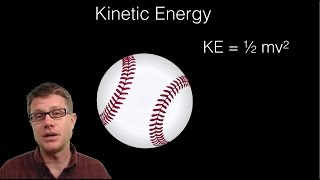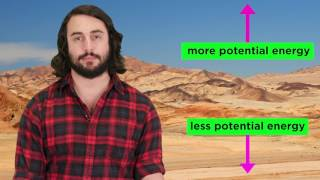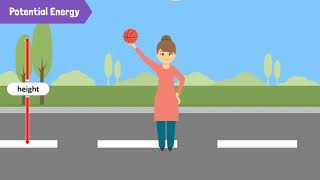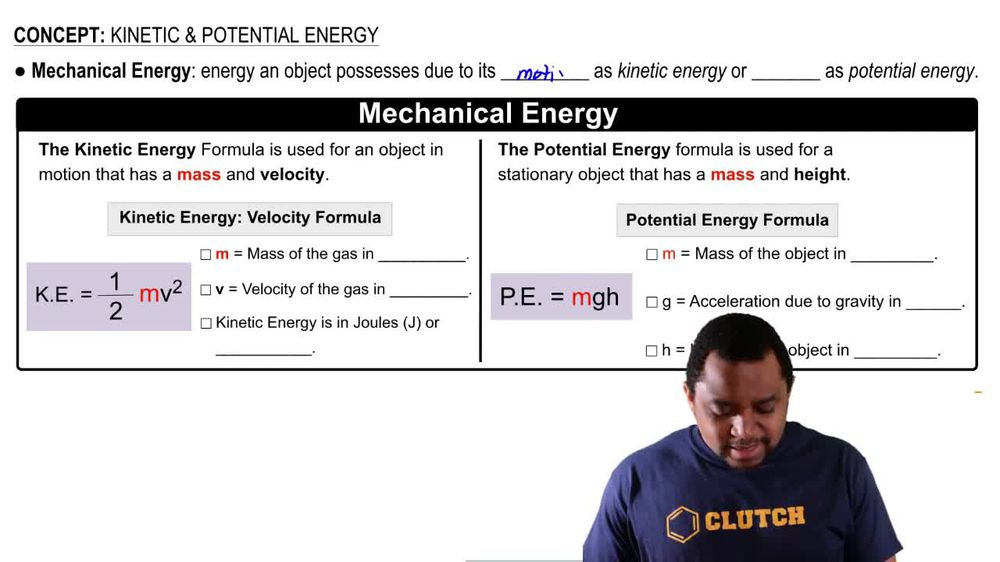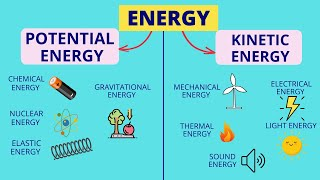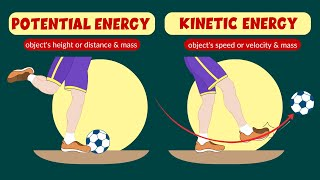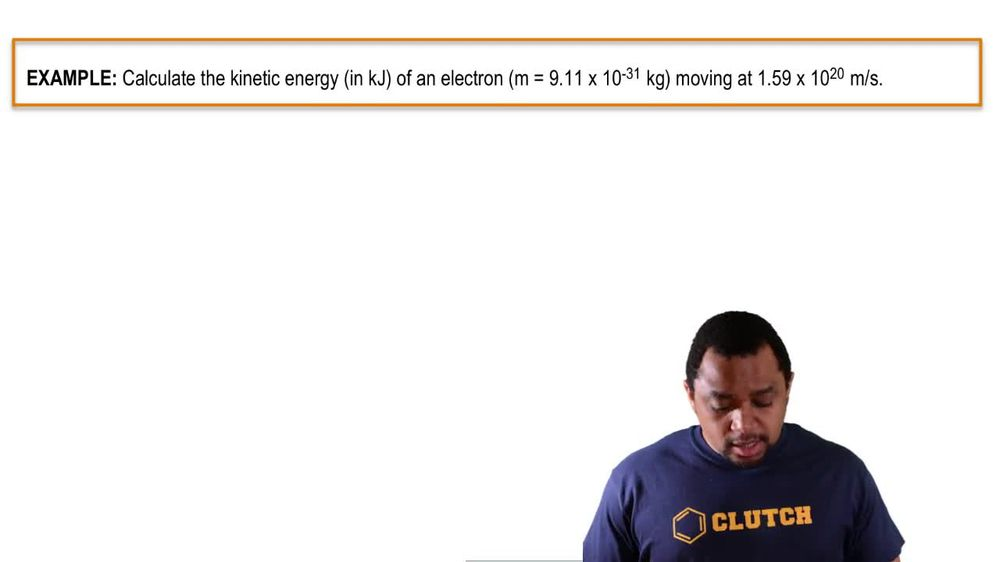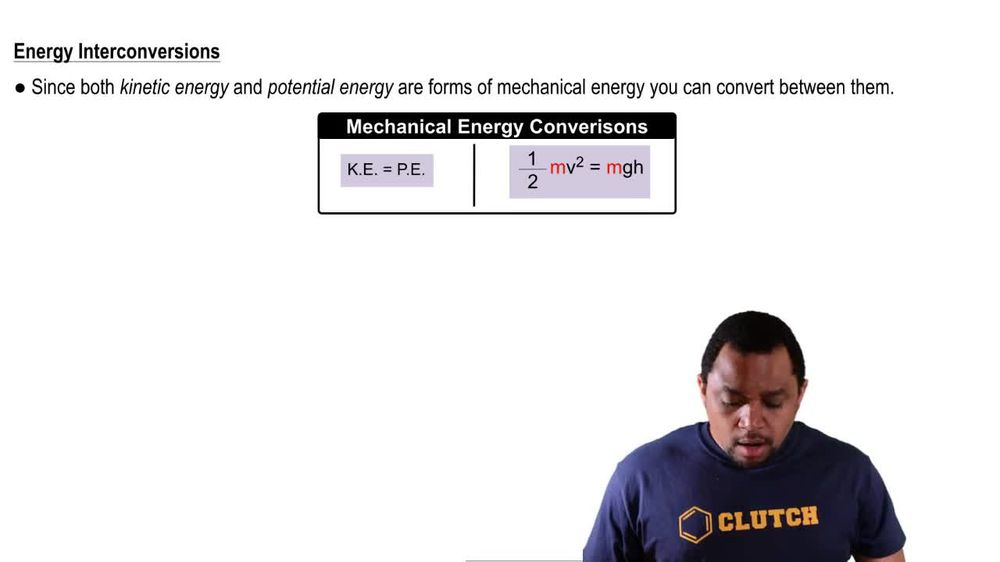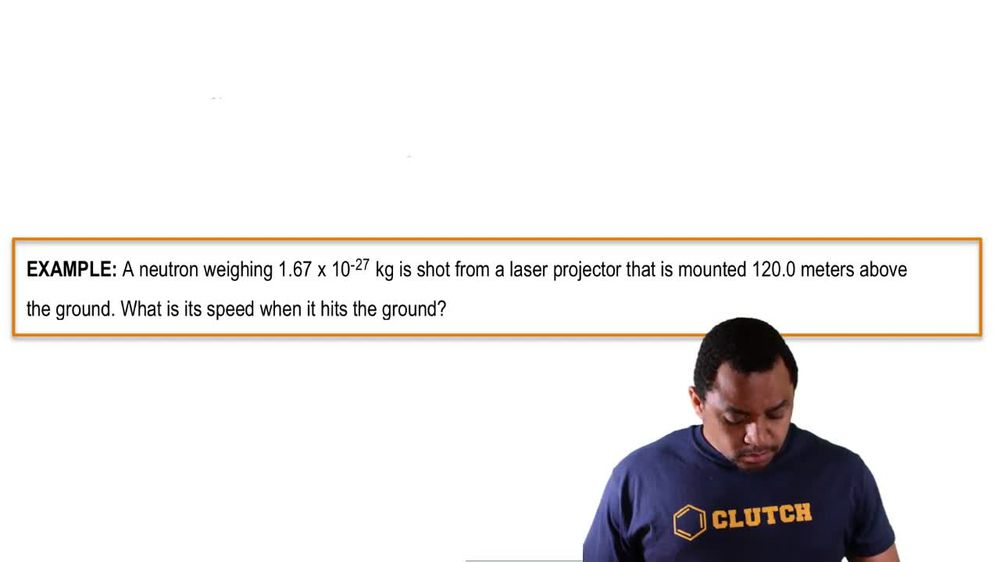Start typing, then use the up and down arrows to select an option from the list.
1. 8. Thermochemistry2. Kinetic & Potential Energy# Kinetic & Potential Energy Example 2

by Jules Bruno
145 views
1
2
1
here. We're told that a neutron way, 1.67 times 10 to the negative 27 kg is shot from a laser projector that has mounted 120 m above the ground. Was it speed when it hits the ground? All right, so we're talking about the neutrons position position initially, and then they're talking about shooting it towards the ground. Therefore, it's converting into kinetic energy. From that information, we should be able to calculate its speed or velocity. Remember, kinetic energy can equal potential energy, since they're both part of mechanical energy. That meat half times M times V square equals M times G times h here, The mass for both would be the same, since it's a neutron that's stationary first and then it's moving. We're looking for velocity or speed, so that V squared is what we're solving for mass again is the same mhm. Then we're going to say gravity do. Acceleration here on earth is 9.8 m over second squared, and it's mounted 120 m above the ground. We're going to multiply these together and multiply everything here together. When we do that, we get 8.35 times 10 to the negative 28. And that's V squared equals 1.96392 times 10 to the negative 24. Then we're gonna divide both sides by 8.35 times 10 to the negative 28. So when we do that, that's gonna isolate R V squared here. So when we do that, we're gonna get the squared equals 2352 And that's gonna be meters squared over seconds squared. Taking the square root of both of those sides, we'll isolate our velocity or speed. So here, when we do that, we get our velocity equal to 48.4974 m over seconds. Here, this has three sig figs. This has four sig figs. So let's just go with the least number Sig figs, so that's gonna be 48.5 meters per second. So that would be the speed or velocity of the neutron as it strikes the ground